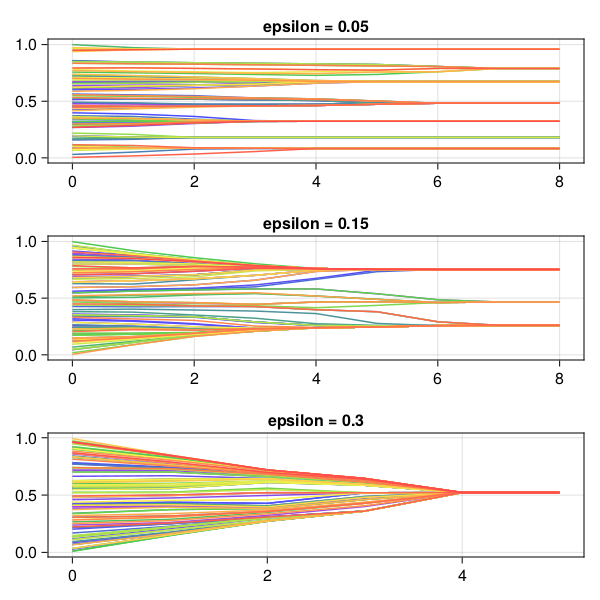# Hegselmann-Krause opinion dynamics

This example showcases

• How to do synchronous updating of Agent properties (also know as Synchronous update schedule). In a Synchronous update schedule changes made to an agent are not seen by other agents until the next step, see also Wilensky 2015, p.286).
• How to terminate the system evolution on demand according to a boolean function.
• How to terminate the system evolution according to what happened on the previous step.

## Model overview

This is an implementation of a simple version of the Hegselmann and Krause (2002) model. It is a model of opinion formation with the question: which parameters' values lead to consensus, polarization or fragmentation? It models interacting groups of agents (as opposed to interacting pairs, typical in the literature) in which it is assumed that if an agent disagrees too much with the opinion of a source of influence, the source can no longer influence the agent's opinion. There is then a "bound of confidence". The model shows that the systemic configuration is heavily dependent on this parameter's value.

The model has the following components:

• A set of n Agents with opinions xᵢ in the range [0,1] as attribute
• A parameter ϵ called "bound" in (0, 0.3]
• The update rule: at each step every agent adopts the mean of the opinions which are within the confidence bound ( |xᵢ - xⱼ| ≤ ϵ).

## Core structures

We start by defining the Agent type and initializing the model. The Agent type has two fields so that we can implement the synchronous update.

using Agents
using Statistics: mean

mutable struct HKAgent <: AbstractAgent
id::Int
old_opinion::Float64
new_opinion::Float64
previous_opinion::Float64
end

There is a reason the agent has three fields that are "the same". The old_opinion is used for the synchronous agent update, since we require access to a property's value at the start of the step and the end of the step. The previous_opinion is the opinion of the agent in the previous step, as the model termination requires access to a property's value at the end of the previous step, and the end of the current step.

We could, alternatively, make the three opinions a single field with vector value.

function hk_model(; numagents = 100, ϵ = 0.2)
model = ABM(HKAgent, scheduler = Schedulers.fastest, properties = Dict(:ϵ => ϵ))
for i in 1:numagents
o = rand(model.rng)
end
return model
end

model = hk_model()
AgentBasedModel with 100 agents of type HKAgent
space: nothing (no spatial structure)
scheduler: fastest
properties: ϵ

Add some helper functions for the update rule. As there is a filter in the rule we implement it outside the agent_step! method. Notice that the filter is applied to the :old_opinion field.

function boundfilter(agent, model)
filter(
j -> abs(agent.old_opinion - j) < model.ϵ,
[a.old_opinion for a in allagents(model)],
)
end

Now we implement the agent_step!

function agent_step!(agent, model)
agent.previous_opinion = agent.old_opinion
agent.new_opinion = mean(boundfilter(agent, model))
end

and model_step!

function model_step!(model)
for a in allagents(model)
a.old_opinion = a.new_opinion
end
end

From this implementation we see that to implement synchronous scheduling we define an Agent type with old and new fields for attributes that are changed via the synchronous update. In agent_step! we use the old field then, after updating all the agents new fields, we use the model_step! to update the model for the next iteration.

## Running the model

The parameter of interest is now :new_opinion, so we assign it to variable adata and pass it to the run! method to be collected in a DataFrame.

In addition, we want to run the model only until all agents have converged to an opinion. From the documentation of step! one can see that instead of specifying the amount of steps we can specify a function instead.

function terminate(model, s)
if any(
!isapprox(a.previous_opinion, a.new_opinion; rtol = 1e-12)
for a in allagents(model)
)
return false
else
return true
end
end

Agents.step!(model, agent_step!, model_step!, terminate)
model
Main.HKAgent(1, 0.2736919294384161, 0.2736919294384161, 0.2736919294384161)

Alright, let's wrap everything in a function and do some data collection using run!.

function model_run(; kwargs...)
model = hk_model(; kwargs...)
agent_data, _ = run!(model, agent_step!, model_step!, terminate; adata = [:new_opinion])
return agent_data
end

data = model_run(numagents = 100)
data[(end-19):end, :]
20×3 DataFrame
Rowstepidnew_opinion
Int64Int64Float64
18810.3209
28820.803089
38830.3209
48840.3209
58850.3209
68860.803089
78870.803089
88880.3209
98890.803089
108900.3209
118910.3209
128920.3209
138930.803089
148940.3209
158950.803089
168960.803089
178970.3209
188980.3209
198990.803089
2081000.803089

Notice that here we didn't speciy when to collect data, so this is done at every step. Instead, we could collect data only at the final step, by re-using the same function for the when argument:

model = hk_model()
agent_data, _ = run!(
model,
agent_step!,
model_step!,
terminate;
when = terminate,
)
agent_data
100×3 DataFrame
Rowstepidnew_opinion
Int64Int64Float64
1710.762829
2720.762829
3730.762829
4740.762829
5750.354652
6760.354652
7770.354652
8780.762829
9790.354652
107100.762829
117110.354652
127120.354652
137130.354652
147140.354652
157150.354652
167160.354652
177170.762829
187180.354652
197190.762829
207200.354652
217210.354652
227220.762829
237230.354652
247240.354652
257250.354652
267260.762829
277270.354652
287280.354652
297290.762829
307300.354652
317310.762829
327320.762829
337330.762829
347340.762829
357350.762829
367360.354652
377370.354652
387380.762829
397390.354652
407400.354652
417410.354652
427420.354652
437430.354652
447440.762829
457450.354652
467460.762829
477470.354652
487480.354652
497490.762829
507500.762829
517510.354652
527520.354652
537530.354652
547540.354652
557550.354652
567560.762829
577570.354652
587580.354652
597590.762829
607600.354652
617610.354652
627620.354652
637630.354652
647640.354652
657650.354652
667660.762829
677670.762829
687680.762829
697690.762829
707700.354652
717710.354652
727720.762829
737730.762829
747740.354652
757750.762829
767760.354652
777770.762829
787780.354652
797790.354652
807800.354652
817810.762829
827820.354652
837830.762829
847840.354652
857850.762829
867860.354652
877870.762829
887880.762829
897890.354652
907900.762829
917910.762829
927920.354652
937930.354652
947940.762829
957950.354652
967960.762829
977970.762829
987980.354652
997990.354652
10071000.762829

Finally we run three scenarios, collect the data and plot it.

using DataFrames, CairoMakie

plotsim(ax, data) =
for grp in groupby(data, :id)
lines!(ax, grp.step, grp.new_opinion, color = cmap[grp.id/100])
end

eps = [0.05, 0.15, 0.3]
figure = Figure(resolution = (600, 600))
for (i, e) in enumerate(eps)
ax = figure[i, 1] = Axis(figure; title = "epsilon = \$e")
e_data = model_run(ϵ = e)
plotsim(ax, e_data)
end
figure